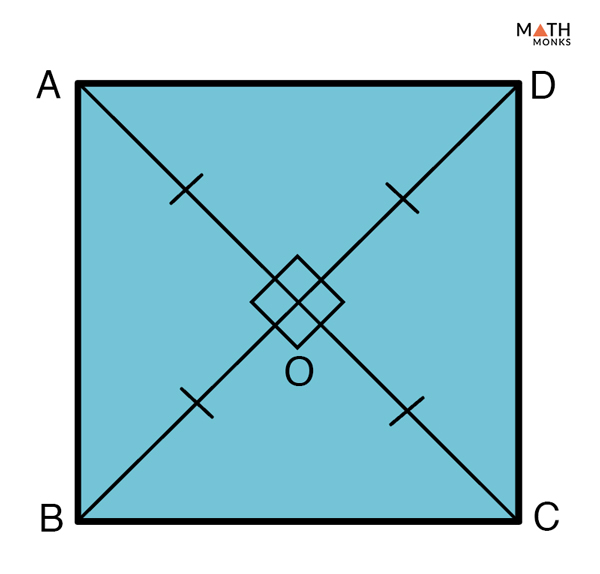## How to Find the Diagonal of a Quadrilateral

Since, a quadrilateral is a four-sided polygon, we can obtain the number of diagonals in a quadrilateral by using the formula given below:

As we know,

The number of diagonals in a polygon = n (n – 3)/2, where n = number of sides of the polygon

For a quadrilateral, n = 4

The number of diagonals in a quadrilateral = 4 (4 – 3)/2

= 4/2

= 2

How Many Diagonals does a Quadrilateral Have

A quadrilateral is thus found to have two diagonals.

## Types of Diagonals in Quadrilateral

Based on their properties, diagonals of a quadrilateral can be of three broad types:

1) Diagonals that Bisect Each Other: Also known as mutually bisecting diagonals, they divide each other into half after they meet.

Let us take the example of a square. After drawing both the diagonals, measure each section of a diagonal. You will find both the sections of a diagonal to be equal. Now measure the other diagonal. You will find the same result. This proves that both the diagonals have bisected each other into half.

Other than a square, a rectangle, a parallelogram, and a rhombus are examples of special quadrilaterals that have diagonals that bisect each other.

2) Perpendicular Diagonals: They are diagonals that intersect each other at right angles (90°). In other words, perpendicular diagonals form four right angles at the point of intersection.

Let us take the example of a rhombus. After drawing both the diagonals, use the edge of a sheet of paper and place them in each one of the four angles. You will find all four angles formed through the intersection of the two diagonals equals 90°. This proves that the two diagonals in a rhombus meet each other at right angles.

Other than a rhombus, a square and a kite are examples of special quadrilaterals that have diagonals that are perpendicular to each other.

3) Perpendicular Bisecting Diagonals: Also known as perpendicular bisectors, they are diagonals that form four right angles (90°) at the point of their intersection. The perpendicular bisecting diagonals divide each other into half after they meet.

Since this property of a diagonal sums up the previous two properties, i.e. the properties of mutually bisecting diagonals and that of the perpendicular bisecting diagonals, any quadrilateral fulfilling the criteria of both the above properties will satisfy the criteria to be a perpendicular bisecting diagonal.

Let us take the example of a square, since its diagonals are both mutually bisecting and intersect at right angles, they are an example of quadrilaterals having perpendicular bisecting diagonals. Similarly, a rhombus is the only other special quadrilateral that has perpendicular bisecting diagonals.

## Diagonals of a Quadrilateral TheoremProve Diagonals of a Quadrilateral Theorem

To prove:

ABCD is a square

Proof:

Procedure: We know a square is a parallelogram with all sides equal and one angle 90°. So, first, we need to prove the given quadrilateral is a parallelogram. Then we should prove whether all its sides are equal with one right angle.
Let ABCD be a quadrilateral, and ‘AC’, ‘BD’ are the two diagonals that bisect each other at ‘O’
Given: AC = BD …… (1)
OA = OC and OB = OD …….. (2)
∠AOB = ∠BOC = ∠COD = ∠AOD = 90° ……. (3)
In △AOB and △COB,
OA = OC … from (2)
∠AOB = ∠COB = 90° … from (3)
OB = OB (Common)
Hence, △AOB ≅ △ COB (By SAS congruence rule)
AB = CB (CPCTC)
Similarly, we can prove,
△AOB ≅ △DOA and AB = CD
△BOC ≅ △COD and CB = DC
Hence, AB = AD = CB = DC
Now, we can write
AB = CD and AD = BC
Thus in quadrilateral ABCD, both the opposite sides are equal.
Hence ABCD is a parallelogram.
As we know, a square is a parallelogram with all sides equal and one right angle 90°.
Now,
In △ABC and △DCB,
AC = BD …. From (1)
AB = DC (Opposite sides of parallelogram are equal)
BC = CB (Common side)
Hence, △ABC ≅ △DCB (By SSS congruence rule)
So, ∠ABC = ∠DCB (CPCTC)
Now, AB ∥ CD (Opposite sides of a parallelogram are parallel), and BC is transversal.
∠B + ∠C = 180° (Interior angles on the same side of transversal is supplementary)
∠B + ∠B = 180° …… from (4)
2∠B = 180°
∠B = 180°/2
= 90°
Thus, ABCD is a parallelogram with all sides equal and one right angle (90°).
(Hence Proved)

## FAQs

Q1. Which quadrilaterals have congruent diagonals?

Ans. A rectangle, a square, and an isosceles trapezoid always have equal or congruent diagonals.

Q2. Which quadrilaterals have diagonals that are not lines of symmetry?

Ans. A rectangle, a parallelogram, and a trapezoid are quadrilaterals that have diagonals that are not lines of symmetry.

Q3. Name a quadrilateral with opposite angles congruent?

Ans. Parallelogram.

Q4. Which quadrilaterals have diagonals of equal length?

Ans. Square and rectangle are quadrilaterals having diagonals that are equal in length.

Q5. How many diagonals does a concave quadrilateral have?

Ans. All quadrilaterals whether convex or concave have two diagonals.